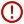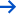Skip to content# Linear Ordinary Differential Equations

• Date Published: December 1997
• availability: This item is not supplied by Cambridge University Press in your region. Please contact Soc for Industrial null Mathematics for availability.
• format: Paperback
• isbn: 9780898713886

## Paperback

### Looking for an inspection copy?

This title is not currently available on inspection

Description
Product filter button
Description
Contents
Resources
Courses
• Linear Ordinary Differential Equations, a text for advanced undergraduate or beginning graduate students, presents a thorough development of the main topics in linear differential equations. A rich collection of applications, examples, and exercises illustrates each topic. The authors reinforce students' understanding of calculus, linear algebra, and analysis while introducing the many applications of differential equations in science and engineering. Three recurrent themes run through the book. The methods of linear algebra are applied directly to the analysis of systems with constant or periodic coefficients and serve as a guide in the study of eigenvalues and eigenfunction expansions. The use of power series, beginning with the matrix exponential function leads to the special functions solving classical equations. Techniques from real analysis illuminate the development of series solutions, existence theorems for initial value problems, the asymptotic behavior solutions, and the convergence of eigenfunction expansions.

## Customer reviews

### Not yet reviewed

Be the first to review

Review was not posted due to profanity

×

## , create a review

(If you're not , sign out)

Please enter a star rating.
Your review must be a minimum of 12 words.

×

## Product details

• Date Published: December 1997
• format: Paperback
• isbn: 9780898713886
• length: 348 pages
• dimensions: 253 x 178 x 15 mm
• weight: 0.636kg
• availability: This item is not supplied by Cambridge University Press in your region. Please contact Soc for Industrial null Mathematics for availability.

Preface. 1. Simple Applications. Introduction
Compartment systems
Springs and masses
Electric circuits
Notes
Exercises
2. Properties of Linear Systems. Introduction
Basic linear algebra
First-order systems
Higher-order equations
Notes
Exercises
3. Constant Coefficients. Introduction
Properties of the exponential of a matrix
Nonhomogeneous systems
Structure of the solution space
The Jordan canonical form of a matrix
The behavior of solutions for large t
Higher-order equations
Exercises
4. Periodic Coefficients. Introduction
Floquet's theorem
The logarithm of an invertible matrix
Multipliers
The behavior of solutions for large t
First-order nonhomogeneous systems
Second-order homogeneous equations
Second-order nonhomogeneous equations
Notes
Exercises
5. Analytic Coefficients. Introduction
Convergence
Analytic functions
First-order linear analytic systems
Equations of order n
The Legendre equation and its solutions
Notes
Exercises
6. Singular Points. Introduction
Systems of equations with singular points
Single equations with singular points
Infinity as a singular point
Notes
Exercises
7. Existence and Uniqueness. Introduction
Convergence of successive approximations
Continuity of solutions
More general linear equations
Estimates for second-order equations
Notes
Exercises
8. Eigenvalue Problems. Introduction
Inner products
Boundary conditions and operators
Eigenvalues
Nonhomogeneous boundary value problems
Notes
Exercises
9. Eigenfunction Expansions. Introduction
Eigenvalues for Green's operator
Convergence of eigenfunction expansions
Extensions of the expansion results
Notes
Exercises
10. Control of Linear Systems. Introduction
Convex sets
Control of general linear systems
Constant coefficient equations
Time-optimal control
Notes
Exercises
Bibliography.

• ## Authors

Earl A. Coddington

Robert Carlson

### Sorry, this resource is locked

Please register or sign in to request access. If you are having problems accessing these resources please email lecturers@cambridge.org

Please note that this file is password protected. You will be asked to input your password on the next screen.

» Proceed

You are now leaving the Cambridge University Press website. Your eBook purchase and download will be completed by our partner www.ebooks.com. Please see the permission section of the www.ebooks.com catalogue page for details of the print & copy limits on our eBooks.

×### Turn stock notifications on?

You must be signed in to your Cambridge account to turn product stock notifications on or off.

Create a Cambridge account×

### Find content that relates to you

This site uses cookies to improve your experience. Close

## Are you sure you want to delete your account?

This cannot be undone.

Thank you for your feedback which will help us improve our service.

If you requested a response, we will make sure to get back to you shortly.

×